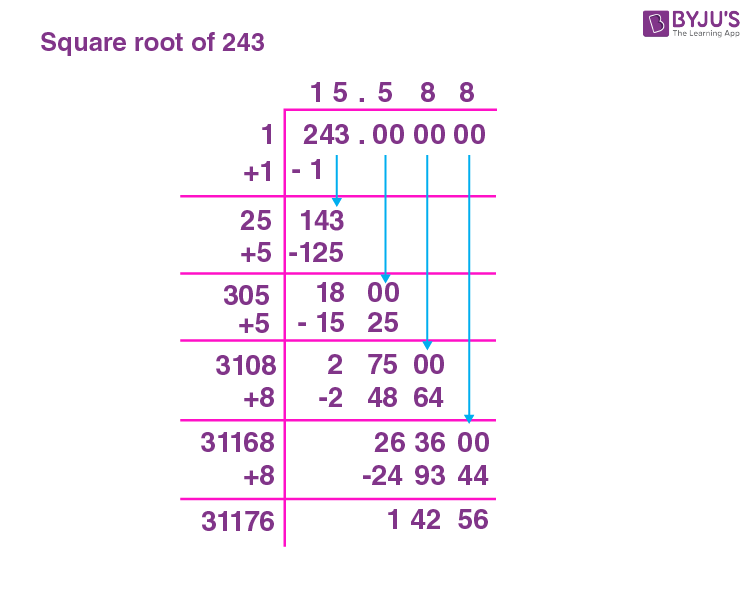# Square Root of 243

The square root of 243 is irrational. To find the square root of a number, the given number should be a perfect square. i,e. a number when multiplied with itself gives a product and that product is a perfect square. When 15 is multiplied by 15, we get 225 or if we multiply 16 with 16 we get 256. The number 243 lies between 225 and 256. Therefore 243 does not have a number, which when multiplied by itself gives 243. Hence 243 is not a perfect square and it does not have a perfect square root. Therefore, √243 is an irrational number. You can refer to irrational numbers.

Note the Following:

• The Square root of 243 = √243 where ‘√’ is the radical, and 243 is the radicand
• Exponential Form of Square root of 243 = 2431/2
• Solution for √243 = 9√3
• Square root of 243 is Irrational = True

## What is the Square root of 243?

The square root of 243 is 9√3, as the square root of 243 is irrational.

 √243 = 9√3

## How to Find the Square root of 243?

There are three methods to find the Square root of 243

• Prime Factorisation method
• Long Division method
• Repeated Subtraction method

### Square root of 243 by Prime Factorisation Method

The Square root of a number can be calculated only if the number is a perfect square number. 243 is not a perfect square number. Let us try to find the simplified solution of √243. Let us start dividing 243 by the smallest prime number, say 3.

The given number, 243 will be expressed as;

 3 243 3 81 3 27 3 9 3 3 × 1

243 = 3 × 3 × 3 × 3 × 3

Grouping into 2 with same divisors:

Group 1 = 3 × 3, considering only 3

Group 2 = 3 × 3, considering only 3

Another divisor 3 is left out as it does not have a pair.

Therefore the square root of 243 = 3 × 3 × √3 = 9√3.

### Square root of 243 by Long Division Method

To understand the long division method, follow the below mentioned detailed steps;

Step 1:  Grouping the given number into pairs

Given number is 243, grouping it as 2 and 43.

 2 43

Step 2: Consider the first number, which is 2.

Let us find a square number that divides 2

i.e

1 × 1 = 1

2 × 2 = 4

The square number that is to be considered for dividing 2 will be 1 x 1

Step 3: Dividing 2 by 1

Step 4: Continue the division using the next number 43

The first number to be used as the next divisor is 1 + 1 = 2 (Divisor of first division + quotient of first division)

Now considering a two digit number starting with 2 to divide 243 will be

21 × 1 = 21

22 × 2 = 44

23 × 3 = 69

24 × 4 = 96

25 × 5 = 125

26 × 6 = 156

Continue the division asSince the Division is incomplete with remainder as eighteen, the square root for the given number 243 will not be a integer. Therefore 243 is an irrational number which is 9√3.

### Square root of 243 by Repeated Subtraction Method.

We found out from the prime factorization method and long division method that 243 does not have a fine square root. Let us try with the repeated subtraction method. Here 243 will be continuously divided by odd numbers starting from 1 till the result ends with zero.

Check out the table below to see if the repeated subtraction results in 0 at any point.

For the given number 243, steps for repeated subtraction are:

 Step 1 243 – 1 = 242 Step 2 242 – 3 = 239 Step 3 239 – 5 = 234 Step 4 234 – 7 = 227 Step 5 227 – 9 = 218 Step 6 218 – 11 = 207 Step 7 207 – 13 = 194 Step 8 194 – 15 = 179 Step 9 179 – 17 = 162 Step 10 162 – 19 = 143 Step 11 143 – 21 = 122 Step 12 122 – 23 = 99 Step 13 99 – 25 = 74 Step 14 74 – 27 = 47 Step 15 47 – 29 = 18 Step 16 18 – 31 = -13

Since the result does not become zero, 243 is not a perfect square number and hence it does not have a perfect square root. The root lies between the numbers 15 and 16, resulting in a decimal root.

## Video Lessons

### Visualising square roots### Finding Square roots## Solved Examples

1. Which number that is lesser than or greater than 243, that has a perfect square root?

225, which is less than 243 and 256 which is greater than 243, are the perfect square numbers. 15 and 16 are their perfect square roots respectively.

2. What is the square root of 289?

17 is square root of 289.

## Frequently Asked Questions on Square root of 243

### What is the Square root of 243?

The Square root of 243 is 9√3, approximately 15.59.

### Does 24 have a square root?

Yes, the square root of 24 is ± 2√6 or 4.898979 (approx.).

### What are the factors of 243?

The factors of 243 are 1, 3, 9, 27, 81 and 243.

### What is 15 square?

15 square is 225.

### Square root of 243 is a rational number. True or False?

False. Square root of 243 is an irrational number.